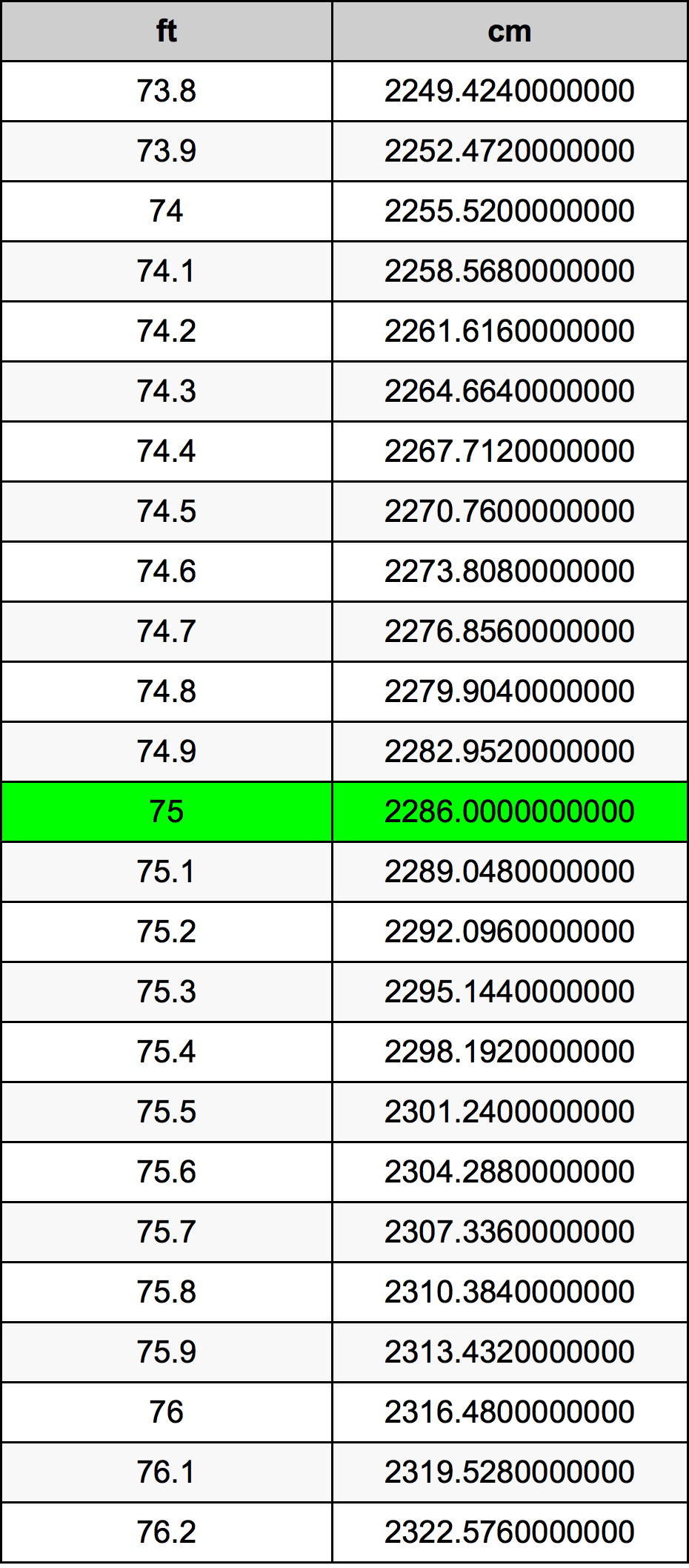Feet To Cm

# 75 ft to cm75 Feet to Centimeters

ft
=
cm

## How to convert 75 feet to centimeters?

 75 ft * 30.48 cm = 2286.0 cm 1 ft
A common question is How many foot in 75 centimeter? And the answer is 2.4606299213 ft in 75 cm. Likewise the question how many centimeter in 75 foot has the answer of 2286.0 cm in 75 ft.

## How much are 75 feet in centimeters?

75 feet equal 2286.0 centimeters (75ft = 2286.0cm). Converting 75 ft to cm is easy. Simply use our calculator above, or apply the formula to change the length 75 ft to cm.

## Convert 75 ft to common lengths

UnitLengths
Nanometer22860000000.0 nm
Micrometer22860000.0 µm
Millimeter22860.0 mm
Centimeter2286.0 cm
Inch900.0 in
Foot75.0 ft
Yard25.0 yd
Meter22.86 m
Kilometer0.02286 km
Mile0.0142045455 mi
Nautical mile0.0123434125 nmi

## What is 75 feet in cm?

To convert 75 ft to cm multiply the length in feet by 30.48. The 75 ft in cm formula is [cm] = 75 * 30.48. Thus, for 75 feet in centimeter we get 2286.0 cm.

## 75 Foot Conversion Table## Alternative spelling

75 ft to Centimeter, 75 ft in Centimeter, 75 Foot to Centimeters, 75 Foot in Centimeters, 75 Feet to Centimeters, 75 Feet in Centimeters, 75 ft to cm, 75 ft in cm, 75 Foot to Centimeter, 75 Foot in Centimeter, 75 Foot to cm, 75 Foot in cm, 75 Feet to Centimeter, 75 Feet in Centimeter